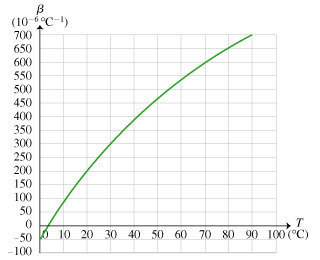# Problem: The anomalous expansion characteristics of liquid water are crucial to many biological systems. Rather than an approximately constant value for the coefficient of volume expansion, the value for water changes drastically, as illustrated in the figure.(A) Below what temperature T does water shrink when heated? Express your answer numerically in degrees Celsius.(B) If the temperature of water at 30°C is raised by 1°C, the water will expand. At approximately what initial temperature T will water expand by twice as much when raised by 1°C? Express your answer numerically in degrees Celsius.

###### FREE Expert Solution

In this problem, we are to explain the consequences of the variables in the thermal expansion equation.

Change in volume:

80% (252 ratings)###### Problem Details

The anomalous expansion characteristics of liquid water are crucial to many biological systems. Rather than an approximately constant value for the coefficient of volume expansion, the value for water changes drastically, as illustrated in the figure.(A) Below what temperature T does water shrink when heated? Express your answer numerically in degrees Celsius.

(B) If the temperature of water at 30°C is raised by 1°C, the water will expand. At approximately what initial temperature T will water expand by twice as much when raised by 1°C? Express your answer numerically in degrees Celsius.